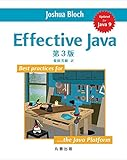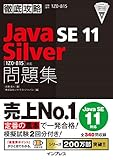Effective Java 第3版Java言語で学ぶデザインパターン入門第3版# Java : Writer - API使用例

Writer (Java SE 17 & JDK 17) の使用例まとめです。
だいたいのメソッドを網羅済みです。
API仕様のおともにどうぞ。

## 概要``````final var path = Path.of("R:", "java-work", "aaa.txt");
System.out.println(path); // R:\java-work\aaa.txt
System.out.println(Files.exists(path)); // false

try (final Writer writer = Files.newBufferedWriter(path)) {

writer.write("abcd");
writer.write("XYZ");
}

System.out.println(str); // abcdXYZ
``````

Writer は使い終わったら close が必要です。
try-with-resources文 を使い、リソースリークが起きないようにしましょう。

※StringWriter など、一部 close しなくても問題ないクラスもあります。

protectedです。

protectedです。

protectedです。

## メソッド

### Writer append (char c)

``````try (final Writer writer = new StringWriter()) {

System.out.println(writer.append('a')); // a
System.out.println(writer.append('b')); // ab
System.out.println(writer.append('X').append('Y').append('Z')); // abXYX
}
``````

### Writer append (CharSequence csq)

``````try (final Writer writer = new StringWriter()) {
final var ret = writer.append("abc").append("XYZ");
System.out.println(ret); // abcXYZ
}
``````
``````try (final Writer writer = new StringWriter()) {

final CharSequence csq1 = "abc";

System.out.println(writer.append(csq1)); // abc

final var sb = new StringBuilder();
final CharSequence csq2 = sb;

sb.append("XY");
System.out.println(writer.append(csq2)); // abcXY

sb.append("Z");
System.out.println(writer.append(csq2)); // abcXYXYZ
}
``````

### Writer append (CharSequence csq, int start, int end)

``````final CharSequence csq = "abcd";

try (final Writer writer = new StringWriter()) {
System.out.println(writer.append(csq, 0, 1)); // a
}

try (final Writer writer = new StringWriter()) {
System.out.println(writer.append(csq, 0, 2)); // ab
}

try (final Writer writer = new StringWriter()) {
System.out.println(writer.append(csq, 0, 3)); // abc
}

try (final Writer writer = new StringWriter()) {
System.out.println(writer.append(csq, 0, 4)); // abcd
}

try (final Writer writer = new StringWriter()) {
//writer.append(csq, 0, 5); // StringIndexOutOfBoundsException
}
``````
``````final CharSequence csq = "abcd";

try (final Writer writer = new StringWriter()) {
System.out.println(writer.append(csq, 0, 4)); // abcd
}

try (final Writer writer = new StringWriter()) {
System.out.println(writer.append(csq, 1, 4)); // bcd
}

try (final Writer writer = new StringWriter()) {
System.out.println(writer.append(csq, 2, 4)); // cd
}

try (final Writer writer = new StringWriter()) {
System.out.println(writer.append(csq, 3, 4)); // d
}
``````

### abstract void close ()

try-with-resources文 を使うのがおすすめです。

``````final var path = Path.of("R:", "java-work", "aaa.txt");
System.out.println(path); // R:\java-work\aaa.txt

try (final Writer writer = Files.newBufferedWriter(path)) {
writer.append("abcd");
}

System.out.println(str); // abcd
``````
``````// try-with-resources文を使わない例です。
final var path = Path.of("R:", "java-work", "aaa.txt");
System.out.println(path); // R:\java-work\aaa.txt

final Writer writer = Files.newBufferedWriter(path);
try {
writer.append("abcd");
} finally {
writer.close();
}

System.out.println(str); // abcd
``````

### abstract void flush ()

``````final var path = Path.of("R:", "java-work", "aaa.txt");
System.out.println(path); // R:\java-work\aaa.txt

try (final Writer writer = Files.newBufferedWriter(path)) {
writer.append("abcd");

writer.flush();
}
``````

### static Writer nullWriter ()

``````final var writer = Writer.nullWriter();

// なにも起きません。
writer.append("abc");
writer.flush();

writer.close();

// closeの後は例外発生
//writer.append("abc"); // IOException: Stream closed
``````

### void write (char[] cbuf)

``````try (final Writer writer = new StringWriter()) {

final char[] cbuf1 = {'a', 'b', 'c'};

writer.write(cbuf1);
System.out.println(writer); // abc

final char[] cbuf2 = {'X', 'Y', 'Z'};

writer.write(cbuf2);
System.out.println(writer); // abcXYZ
}
``````

### abstract void write (char[] cbuf, int off, int len)

``````final char[] cbuf = {'a', 'b', 'c', 'd'};

try (final Writer writer = new StringWriter()) {
writer.write(cbuf, 0, 1);
System.out.println(writer); // a
}

try (final Writer writer = new StringWriter()) {
writer.write(cbuf, 0, 2);
System.out.println(writer); // ab
}

try (final Writer writer = new StringWriter()) {
writer.write(cbuf, 0, 3);
System.out.println(writer); // abc
}

try (final Writer writer = new StringWriter()) {
writer.write(cbuf, 0, 4);
System.out.println(writer); // abcd
}

try (final Writer writer = new StringWriter()) {
//writer.write(cbuf, 0, 5); // IndexOutOfBoundsException
}
``````
``````final char[] cbuf = {'a', 'b', 'c', 'd'};

try (final Writer writer = new StringWriter()) {
writer.write(cbuf, 0, 4);
System.out.println(writer); // abcd
}

try (final Writer writer = new StringWriter()) {
writer.write(cbuf, 1, 3);
System.out.println(writer); // bcd
}

try (final Writer writer = new StringWriter()) {
writer.write(cbuf, 2, 2);
System.out.println(writer); // cd
}

try (final Writer writer = new StringWriter()) {
writer.write(cbuf, 3, 1);
System.out.println(writer); // d
}
``````

### void write (int c)

``````try (final Writer writer = new StringWriter()) {

writer.write('a');
System.out.println(writer); // a

writer.write('b');
System.out.println(writer); // ab

writer.write('X');
System.out.println(writer); // abX

writer.write('Y');
System.out.println(writer); // abXY

writer.write('Z');
System.out.println(writer); // abXYZ
}
``````

### void write (String str)

``````try (final Writer writer = new StringWriter()) {

writer.write("abc");
System.out.println(writer); // abc

writer.write("XYZ");
System.out.println(writer); // abcXYZ
}
``````

### void write (String str, int off, int len)

``````final var str = "abcd";

try (final Writer writer = new StringWriter()) {
writer.write(str, 0, 1);
System.out.println(writer); // a
}

try (final Writer writer = new StringWriter()) {
writer.write(str, 0, 2);
System.out.println(writer); // ab
}

try (final Writer writer = new StringWriter()) {
writer.write(str, 0, 3);
System.out.println(writer); // abc
}

try (final Writer writer = new StringWriter()) {
writer.write(str, 0, 4);
System.out.println(writer); // abcd
}

try (final Writer writer = new StringWriter()) {
//writer.write(str, 0, 5); // IndexOutOfBoundsException
}
``````
``````final var str = "abcd";

try (final Writer writer = new StringWriter()) {
writer.write(str, 0, 4);
System.out.println(writer); // abcd
}

try (final Writer writer = new StringWriter()) {
writer.write(str, 1, 3);
System.out.println(writer); // bcd
}

try (final Writer writer = new StringWriter()) {
writer.write(str, 2, 2);
System.out.println(writer); // cd
}

try (final Writer writer = new StringWriter()) {
writer.write(str, 3, 1);
System.out.println(writer); // d
}
``````